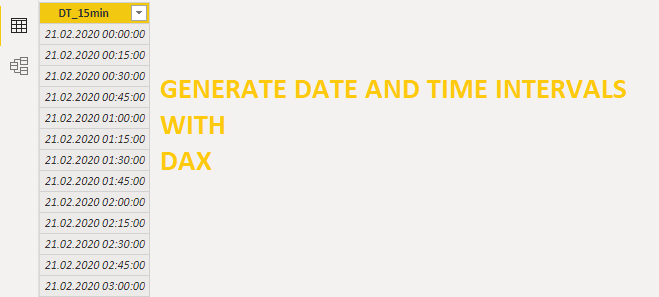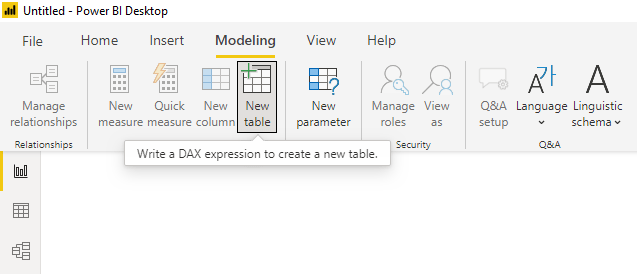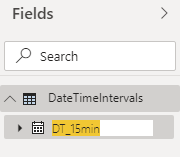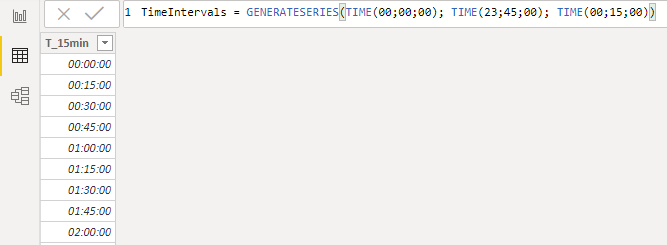# How to generate date and time interval list with DAXHere is how to create a new table and generate a dynamic date and time interval list with DAX. In this example, I will build a table with a column that contains 15 minute intervals for the last 7 days.

To do that, I will use functions GENERATESERIES, TODAY  and principle “Date and time are just numbers”.

GENERATESERIES requires a few simple arguments.

`GENERATESERIES(<startValue>, <endValue>[, <incrementValue>])`

In this case – start date, end date and 15 minute interval as a value for incrementValue argument.

## Date and time are just numbers

If we look at the 15-minute interval as a fraction of the day, then we can calculate that by using the same logic as in Excel. 15 minute interval equals 1 divided by 24 hours and divided with 4. Because in one hour we have 4 15 minute intervals.

## Create DAX table with date and time interval column

First of all, go to the modeling tab and create a new table.Give a name to the table and use GENERATESERIES to create a date and 15 minute interval list for the last 7 days. I don’t need the last 15 minute interval, and that’s why I subtracted one interval from the <endValue>.

You might have different argument separators.

`DateTimeIntervals = GENERATESERIES(TODAY() - 7; TODAY() - 1/24/4; 1/24/4)`Go to the field list and rename that column as you wish.With the DATE function, you can create a static list of dates and times.

`DateTimeIntervals2 = GENERATESERIES(DATE(2020;2;1); DATE(2020;2;29) - 1/24/4; 1/24/4)`Or just a TIME function to create a static list of time intervals. As you can see, it is possible to define incrementation with the TIME function.

`TimeIntervals = GENERATESERIES(TIME(00;00;00); TIME(23;45;00); TIME(00;15;00))`Take a look at this other post on how to generate time and date intervals with Power Query.

Special thanks to Muhammad for the question that was an inspiration to create this post.

### 3 comments on “How to generate date and time interval list with DAX”

1.•2.